Share filter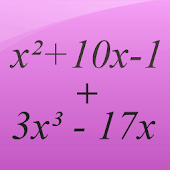Developed by in Education category

3/5.0 Ratings

50,000 - 100,000 Installs

791 Reviews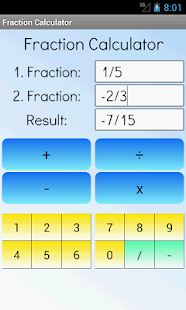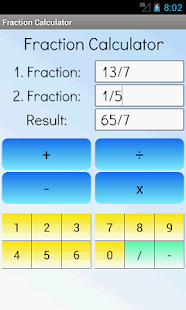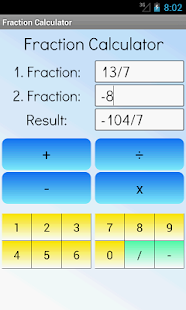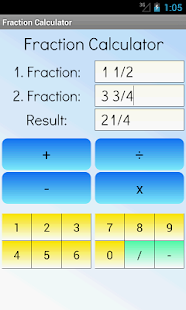### Description

This free math calculator lets you add, subtract, multiply and divide fractions. You can use common fractions (like 1/2) and mixed fractions (like 2 4/5).

Best mathematical tool for school and college! If you are a student, it will helps you to learn arithmetics and calculations with fraction numbers.

Note: A common fraction consists of an integer numerator, displayed above a line (or before a slash in this app), and a non-zero integer denominator, displayed below (or after the slash) that line. Numerators and denominators are also used in fractions that are not common, including compound fractions, complex fractions, and mixed numerals.

This content has been crawled through Play Store, we don't store any APK file.